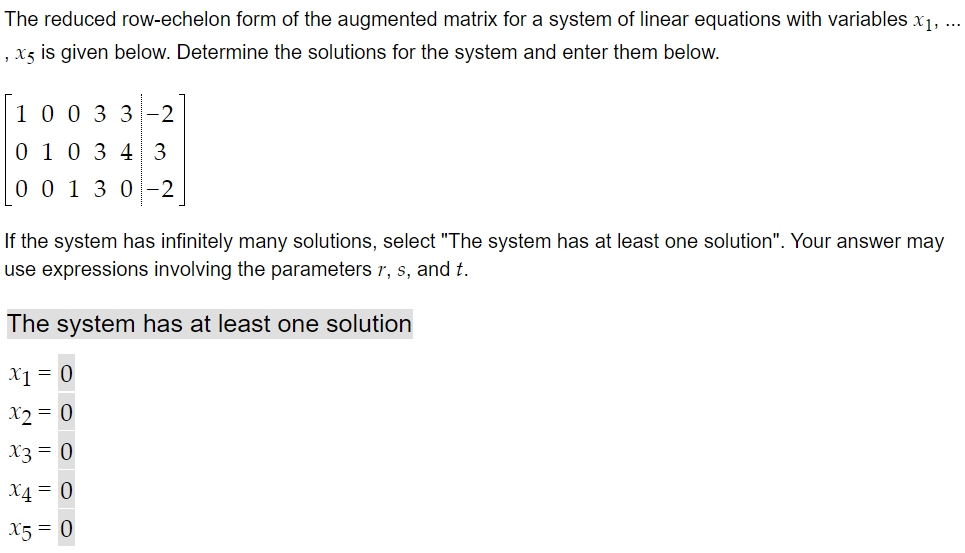# Question Solved1 AnswerThe reduced row-echelon form of the augmented matrix for a system of linear equations with variables X1, ... , x5 is given below. Determine the solutions for the system and enter them below. 1 0 0 3 3 -2 0 1 0 3 4 3 0 0 1 3 0-2 If the system has infinitely many solutions, select "The system has at least one solution". Your answer may use expressions involving the parameters r, s, and t. The system has at least one solution X1 = 0 x2 = 0 X3 = 0 X4 = 0 15= 0Transcribed Image Text: The reduced row-echelon form of the augmented matrix for a system of linear equations with variables X1, ... , x5 is given below. Determine the solutions for the system and enter them below. 1 0 0 3 3 -2 0 1 0 3 4 3 0 0 1 3 0-2 If the system has infinitely many solutions, select "The system has at least one solution". Your answer may use expressions involving the parameters r, s, and t. The system has at least one solution X1 = 0 x2 = 0 X3 = 0 X4 = 0 15= 0
More
Transcribed Image Text: The reduced row-echelon form of the augmented matrix for a system of linear equations with variables X1, ... , x5 is given below. Determine the solutions for the system and enter them below. 1 0 0 3 3 -2 0 1 0 3 4 3 0 0 1 3 0-2 If the system has infinitely many solutions, select "The system has at least one solution". Your answer may use expressions involving the parameters r, s, and t. The system has at least one solution X1 = 0 x2 = 0 X3 = 0 X4 = 0 15= 0